# Accurate analysis of harmonic Hall voltage measurement for spin–orbit torques

## Abstract

An accurate method is developed to extract the spin–orbit (SO) effective fields by analyzing harmonic Hall voltage measurements and deriving detailed analytical equations that consider both the z component of the applied magnetic field and the second-order perpendicular magnetic anisotropy. The method is tested by analyzing the results of a macrospin simulation. The SO effective fields that are extracted from the analysis are consistent with the input SO effective fields that are used in the macrospin simulation over the entire range of the polar magnetization angle and for a wide range (0–2) of the ratio of the planar to the anomalous Hall voltage considered in this study. The accuracy of the proposed method is demonstrated via a systematic study that involves comparing its results with those of a conventional analytical method.

## Introduction

In-plane currents in a nonmagnetic (NM)/ferromagnetic (FM) bilayer nanostructure can generate a torque due to the spin–orbit (SO) coupling, which is known as the SO torque (SOT). SOT is sufficient for reversing the magnetization in the FM layer.1 Numerous studies have been conducted to identify the principal mechanism of the SOT as either the spin Hall effect (SHE) in the NM layer2, 3, 4 or the interfacial SO coupling—frequently referred to as the Rashba effect—at the NM/FM interface.5, 6, 7, 8, 9, 10, 11, 12 In a system in which the NM/FM interface is perpendicular to the z axis and the in-plane currents flow along the x axis, spin currents that are polarized along the y axis are generated in the system based on the SHE induced by a bulk SO coupling in the NM layer. The spin currents are injected into the adjacent FM layer, which causes torque to be transferred to the magnetization of the FM layer. The SHE-induced SOT generates a strong damping-like torque (TDLm × m × y) and a weak field-like torque (TFLm × y).13, 14 Theoretically, the strength of the SHE-induced SOT is known to be independent of the magnetization direction of the FM layer. For an interfacial SO coupling-induced SOT, spins that are polarized along the y axis accumulate due to the broken inversion symmetry at the NM/FM interface. Direct exchange coupling between the magnetization of the FM layer and the accumulated spins generates a strong TFL but a weak TDL.7, 15, 16, 17, 18 Unlike the strength of the SHE-induced SOT, the interfacial SO coupling-induced SOT is known to depend on the magnetization direction of the FM layer.19, 20, 21 In these two cases, both the SHE and the interfacial SO coupling qualitatively induce the same torque on the FM layer. To identify the dominant mechanism of the SOT, a quantitative analysis of the values of TDL and TFL over an extensive range of magnetization angles is required.19, 20, 21

The harmonic Hall voltage measurement method is a useful approach for quantifying the effective fields of TDL and TFL that originate from the SOT.22 This method is particularly suitable for identifying the angular dependence of the SOT that acts on the FM layer with perpendicular magnetization.19, 20 Several corrections are required for an accurate analysis of the measured results, including the measured results for the planar Hall effect (PHE),20, 23 the out-of-plane component of the external magnetic field20 and the anomalous Nernst effect.20, 24 In the harmonic Hall voltage measurement, the second harmonic voltage (V2ω) consists of two major components: anomalous Hall voltage and planar Hall voltage (denoted as VAHE and VPHE, respectively).20, 23 When an external magnetic field (Hext) is applied along the longitudinal (x) direction, the V2ω values from the AHE and PHE are proportional to TDL and TFL, respectively. Under a transverse (y) Hext, however, these values are proportional to TFL and TDL, respectively. This necessitates the use of an analytical solution that is based on Cramer’s rule to separate TFL and TDL.23 This analytical solution has been successfully applied to only a system in which VPHE<VAHE. For a system in which VPHE>VAHE, such as the W/CoFeB/MgO trilayer structure,25 a divergence occurs in the solution, which makes it extremely difficult to analyze the measurement results.

This problem can be overcome by incorporating some necessary corrections into the analysis of the measurement results, including measurement results for the out-of-plane component of Hext. As coherent magnetization rotation is an important requirement in the analysis of harmonic Hall voltage measurement results, Hext is usually applied along the direction tilted slightly (4°−15°) from the basal plane (x–y plane).20 In this condition, the z component of Hext has a nonzero value, although it has been neglected to simplify the analysis. This assumption is reasonable in the low-Hext range, where the magnetization direction is close to the z axis and the perpendicular magnetic anisotropy (PMA) field is consequently dominant over the z component of Hext.23 The simplifying assumption, however, is not valid in the high-Hext range, where the magnetization direction deviates considerably from the z axis, with a resultant reduction in the PMA field. As a result, the PMA field loses its dominance over the z component of Hext. Several attempts have been made to include the z component of Hext, which is obtained by repetitively solving equilibrium torque equations until the desired convergence is achieved (the recursive method).19, 20 However, this method is complicated, and it has not been validated for systems with VPHE>VAHE. Unwanted voltages, which originate from thermoelectric effects such as the anomalous Nernst effect, should be eliminated from the harmonic signals. Although several methods have been proposed for this purpose,20, 24 erasing all artificial signals remains difficult. Another important issue that has not been considered is the inclusion of the second-order PMA in an analysis of the harmonic Hall voltage measurement results. The inclusion of the second-order PMA is considered important because many PMA materials exhibit second-order PMA, whose strength is comparable to that of the first-order PMA in many cases.26, 27

In this study, two corrections—one for the z component of Hext and the other for the second-order PMA—are considered in the analysis of the harmonic Hall voltage measurement results. All related analytical equations are described. Both conventional and refined analytical methods are used to analyze the results of the macrospin simulation, which mimics the harmonic Hall voltage measurement by numerically solving the Landau–Lifshitz–Gilbert equation.28, 29 The accuracy of these two analytical methods is tested by comparing the input SO effective fields for the macrospin simulation with the input SO effective fields calculated by analytical methods. To test the refined analytical method, systems are examined over a wide ratio R, which is defined as VPHE/VAHE. A similar comparative study that analyzes the experimental results of harmonic Hall voltage measurements for a Pt/Co/MgO structure was also performed.

## Materials and methods

### Analytical solutions for conventional approach

When an in-plane AC current with frequency ω (IAC=I0 sinωt) is applied to an NM/FM bilayer structure, the angle between the z axis and the magnetization of the FM layer (θM) and the angle between the x axis and the orthographic projection of the magnetization on the xy plane (ϕM) oscillate as θM(t)=θM°+ΔθM sinωt and ϕM(t)=ϕM°+ΔϕM sinωt. Here the superscript ° and the symbol Δ denote the value in the absence of IAC and the amplitude of the related angles, respectively. The total energy equation for the NM/FM bilayer structure can be expressed as follows: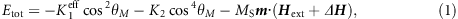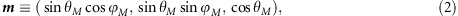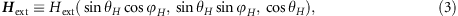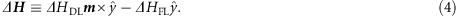Here K1eff is the effective first-order PMA energy density that considers the demagnetizing term, that is, K1eff=K1NdMS2/2 (K1, Nd and MS are the first-order PMA energy density, demagnetizing factor and saturation magnetization, respectively).30 K2 is the second-order PMA energy density.30 m is the unit vector of magnetization. The effective magnetic field (ΔH) induced by the maximum value of the in-plane AC current (I0) is composed of the damping-like effective field (ΔHDL) and the field-like effective field (ΔHFL). θH and ϕH are the polar angle and azimuthal angle of Hext, respectively. Given that the in-plane anisotropy is negligibly small over the PMA field, ϕM° is assumed to be identical to ϕH. The values of ΔθM and ΔϕM can be analytically expressed as follows (refer to Supplementary Equations (S1)–(S13) for a detailed derivation):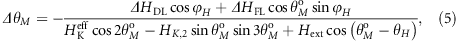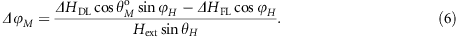Here HKeff and HK1eff are the effective PMA field and the effective first-order PMA field, respectively, and HK,2 is the second-order PMA field. These parameters are defined as follows: HKeffHK,1eff+HK,2; HK,1eff≡2K1eff/MS; HK,2≡4K2/MS. Note that Equations (5) and (6) are identical to the analytical expressions derived by Hayashi et al.23 when HK,2=0. If the values of ΔθM and ΔϕM are sufficiently small, the components of the m vector can be approximated in the form m(t)=m°+(2Δm) sinωt: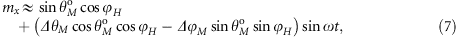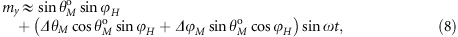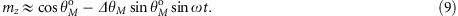Both the anomalous Hall voltage and planar Hall voltage contribute to the measured Hall voltage: VH=IAC RH=IAC RAHE mz+IAC RPHE mx my.20, 31, 32 Here RAHE and RPHE are the anomalous and planar Hall resistance, respectively. With the application of IAC, the m values oscillate as given in Equations (7)–(9) with the resultant expressions for Hall voltages as follows: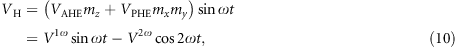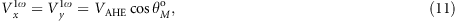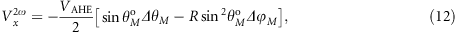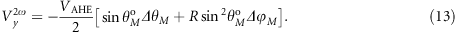Here the following relations exist: VAHE=I0 RAHE and VPHE=I0 RPHE. The subscripts x and y indicate that the harmonic Hall voltages are measured at ϕH=0° and ϕH=90°, respectively. The first harmonic Hall voltage (V1ω) contains information about the θM° value, and the second harmonic Hall voltage (V2ω) contains information about the ΔθM and ΔϕM values. The conventional analytical solution considers only the case in which the magnetization direction has slightly deviated from the z axis (θM°≈0°). In this case, the z component of Hext is negligibly small over the PMA field along the same direction (Hext cosθH HKeff cosθM°), and therefore, the assumption of sinθM°=Hext/HKeff in the conventional solution is reasonable.8, 23, 33 Note that the contribution due to HK,2 is also negligible at θM°≈0° (Equation (5)). With this assumption, V1ω and V2ω can be rewritten as follows: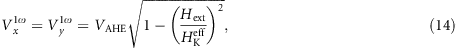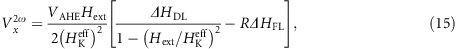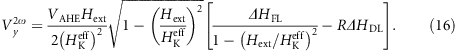The second harmonic Hall voltages, as given in Equations (15) and (16), are composed of two terms that contain ΔHDL and ΔHFL. When the R ratio is negligibly small, the values of ΔHDL and ΔHFL can be obtained using the following T ratios: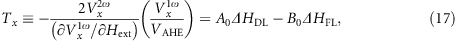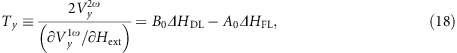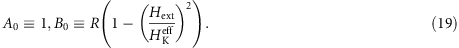At R=0, the values of Tx and Ty are identical to the values of ΔHDL and −ΔHFL, respectively.8 When the R ratio becomes comparatively large, Tx and Ty should be corrected using Cramer’s rule.23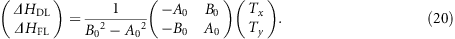In Equation (20), ΔHDL and ΔHFL can be calculated if the determinant B02A02 is not 0. If B02A02 is 0, it is not possible to obtain the individual values of ΔHDL and ΔHFL; it is only possible to obtain the relation Tx=TyHDL−ΔHFL (Equations (17)–(19)).

### Analytical solutions for refined approach

The assumption of Hext cosθH HKeff cosθM° is not valid at high Hext values. In this case, the θH value is not negligible, and ΔθM and ΔϕM (Equations (5) and (6)) must be substituted into Equations (12) and (13) to obtain the expression for V2ω: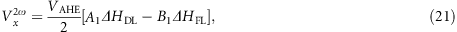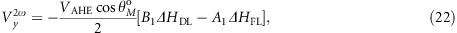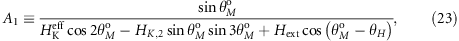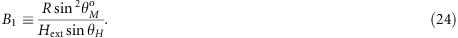Considering that Vx1ω=Vy1ω=VAHE cosθM° (Equation (11)), the G ratios, which correspond to the T ratios in the conventional approach, can be defined as follows: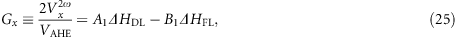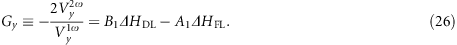Similar to the conventional analytical equations, the refined equations also need to be solved using Cramer’s rule (as given in Equation (20)).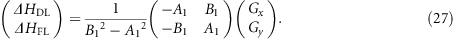## Results

### Conventional analysis

The conventional analytical method is used to analyze the results of the macrospin simulation. Figure 1a and b shows the results for V1ω as a function of Hext in two different systems: (a) HK,1eff=5 kOe and HK,2=0 kOe; and (b) HK,1eff=5 kOe and HK,2=−1 kOe. Two sets of results are shown in Figure 1a and b: the first set is obtained from the macrospin simulation (squares), and the second set is obtained from Equation (14), which is based on the conventional assumption of sinθM°=Hext/HKeff (dashed lines). For the macrospin simulation, the following parameters are applied: ΔHDL=−50 Oe; ΔHFL=−100 Oe; θH=86°; and VAHE=1 mV. Refer to Supplementary Figure S1 for a detailed description of the macrospin simulation. Agreement between the results obtained from the macrospin simulation and the results obtained from Equation (14) based on the conventional analytical method, which is the focus of this study, is only obtained in the low-Hext range. In the high-Hext range, the deviation is very large, which indicates the limited validity of conventional solutions.

Figure 1c and d shows the analytical results for B02A02 calculated from Equation (19) as a function of Hext at two different R values of 0.3 (red lines) and 1.75 (blue lines) (R=VPHE/VAHE). The results in Figure 1c pertain to the system with HK,1eff=5 kOe and HK,2=0, and the results in Figure 1d pertain to the system with HK,1eff=5 kOe and HK,2=−1 kOe. The HKeff values for both systems are also indicated in the figures. The detailed equation for B02A02 is rewritten for clarity: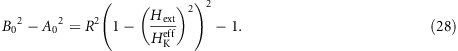Recalling that Hext/HKeff is approximated with sinθM°, we note that the results for B02A02 at Hext>HKeff have no physical meaning. According to Equation (28), the B02A02 value decreases from R2−1 to −1 as the Hext value increases from 0 to HKeff. The determinant B02A02 always has a negative value at R<1. At R1, however, the determinant can have both positive values and negative values over the Hext range of 0–HKeff, which indicates the occurrence of a crossover (B02A02=0) at a certain Hext value. This feature is visible in the results shown in Figure 1c and d. In both systems, that is, with HK,2=0 and −1 kOe, the B02A02 value is always negative at R=0.3; at R=1.75, it initially has a positive value, after which it passes through 0 and then has a negative value. The crossovers occur at 3.3 and 2.6 kOe for the systems with HK,2=0 kOe and HK,2=−1 kOe, respectively. Recalling that Tx=TyHDL−ΔHFL when the determinant is 0, an Hext value should exist at Tx=Ty when R>1.

Figure 2a–f shows the results for Vx2ω and Vy2ω (a and b) and Tx and Ty (c and d) as functions of Hext and the results for ΔHDL and ΔHFL (e and f) as functions of θM° for two different systems, that is, with HK,2=0 (solid lines) and HK,2=−1 kOe (dashed lines). The left (Figure 2a, c and e) and right panels (Figure 2b, d and f) show the results for R=0.3 and 1.75, respectively. The results for V2ω were obtained by the macrospin simulation, and the results for Tx and Ty (Equations (17) and (18)) and ΔHDL and ΔHFL (Equation (20)) were analytically calculated using the simulation results. As shown in Figure 2a and b, the sign of Vx2ω for a small R value of 0.3 is negative, whereas the sign of Vx2ω for a large R value of 1.75 is positive. Of the two major contributions of VAHE and VPHE to V2ω, the sign of the former is negative, but that of the latter is positive. Equations (15) and (16) predict this behavior (the opposite signs of VAHE and VPHE) and explain that the Vy2ω value for R=1.75 is higher than that for R=0.3.

The results for Vx2ω and Vy2ω and their variation with R have a critical effect on Tx and Ty. At R=0.3, the signs of Tx and Ty are opposite because the signs of Vx2ω and Vy2ω are the same, indicating that there are no Hext values at which Tx=Ty in both systems, that is, for HK,2=0 kOe and HK,2=−1 kOe (Figure 2c). These results are consistent with the results for B02A02 (Figure 1c and d). The values of Tx and Ty are the same at a specific Hext value, at which B02A02=0 (Equation (19)). For R=1.75, the signs of Tx and Ty are the same because the signs of Vx2ω and Vy2ω are opposite (Figure 2d). There are Hext values for which Tx=Ty exist in both systems, that is, with HK,2=0 kOe and HK,2=−1 kOe. The positions, however, differ from those for which B02A02=0. The Hext values in the former case are 3.6 and 3.8 kOe for the systems with HK,2=0 and HK,2=−1 kOe, respectively, whereas those in the latter case are 3.3 and 2.6 kOe, respectively. These deviations occur because the determinant poorly reflects the behavior of the first harmonic.

The inappropriate determinant, that is, B02A02, causes large errors in the SO effective fields, as shown in Figure 2e and f. Recalling that the input SO effective fields are ΔHDL=−50 Oe and ΔHFL=−100 Oe, we consider the results at R=0.3 (Figure 2e) to be reliable in the θM° range from 0° to the angles that correspond to Hext=HKeff. These angles are 61° and 52° when HK,2=0 kOe and HK,2=−1 kOe, respectively. Beyond these two angles, which are indicated by the vertical and horizontal lines in Figure 2e and f, respectively, the output SO effective fields start to deviate from the input values. The indicated regions end not at 90° but at ~82° as the m vector is not completely aligned along the x or y axis, even for Hext=10 kOe (Figure 1a and b). The output SO effective fields reveal a divergence, which is physically meaningless at θM°=~81° (HK,2=0 kOe) and ~75° (HK,2=−1 kOe). The deviations are very large at R=1.75 (Figure 2f). For the system with HK,2=0 kOe, divergence occurs even at ~37°, which is not located in the region of physical insignificance (indicated by vertical lines). A similar behavior is observed for the system with HK,2=−1 kOe, where the divergence occurs at ~32°. These divergences are attributed to the misallocation of the Hext value, at which B02A02=0 (Figure 1c and d). The occurrence of the additional divergences significantly limits the reliability of the conventional analytical method for both systems, that is, with HK,2=0 kOe and HK,2=−1 kOe, as shown in Figure 2f.

### Refined analysis

The main reason behind the unreliable results obtained from the conventional analysis is the determinant, which poorly describes the behavior of the first harmonic Hall voltage. For an accurate evaluation of the determinant, the refined analysis begins by determining the relation between θM° and Hext, which can be obtained using the equation θM°=cos−1(V1ω/VAHE) or the total energy equation34 (Equation (1)). The results for V1ω as a function of Hext (Figure 1a and b) can be applied to obtain the relation. Figure 3a and b shows the results for the determinant B12A12 as a function of the Hext obtained from the refined analysis (Equations (23) and (24)) for the systems with HK,2=0 kOe and HK,2=−1 kOe, respectively. The solid lines in Figure 3a and b indicate the results for B12A12, as calculated using HK,2=0 and HK,2=−1 kOe from the macrospin simulation and the refined analysis, respectively. Using the relation between θM° and Hext in the refined analysis enables the behavior of V1ω to be duly reflected in the determinant. At R=1.75, the Hext values for which B12A12=0 are 3.6 and 3.8 kOe for the systems with HK,2=0 and HK,2=−1 kOe, respectively; these Hext values are identical to those obtained at Tx=Ty (Figure 2d). To apply the behavior of V1ω to the determinant, the determinant B12A12 needs to be calculated with a precise value of HK,2, which was used in the macrospin simulation. To demonstrate the importance of the inclusion of HK,2, the determinants were also calculated by disregarding HK,2 (even though the actual HK,2 value of the system is −1 kOe); these results are also shown in Figure 3b (dotted lines). The difference between the two cases of R=0.3 and 1.75 is very large, which indicates that HK,2 should be considered in the analysis. At R=1.75, for example, the Hext value for which the determinant is 0 is misallocated from 3.8 to 3.2 kOe when HK,2 is disregarded; a new location that reveals a 0 value of the determinant emerges at Hext=9.0 kOe.

Figure 4a and b shows the results for Gx and Gy, which correspond to Tx and Ty in the conventional analysis at R=0.3 and 1.75, respectively. The results for the systems with HK,2=0 (solid lines) and HK,2=−1 kOe (dashed lines) are shown. Note the Hext values for which Gx=Gy are 3.6 and 3.8 kOe for the systems with HK,2=0 kOe and HK,2=−1 kOe, respectively. These Hext values are identical to the Hext values for which the determinant B12A12=0 (Figure 3a and b). This finding contradicts the case of the conventional analysis, where the Hext value for which the determinant is 0 differs substantially from the Hext value for which Tx=Ty (Figures 1c and d, and 2d,). With the new set of results for the determinant and the G ratios, the task of calculating the SO effective fields is straightforward; these results as a function of θM° are shown in Figure 4c and d, for R=0.3 and 1.75, respectively. Two sets of results are shown: one set for the system with HK,2=0 kOe (solid lines) and one set for the system with HK,2=−1 kOe (dashed lines). As shown in Figure 4c and d, in both systems, the calculated values of ΔHDL and ΔHFL are consistent with the input values for the macrospin simulation (over the entire θM° range of 0°–~82°); this finding demonstrates the reliability of the refined analysis. At R=0.3, the agreement between the two systems is perfect to such an extent that the solid lines for the HK,2=0 kOe system completely overlap with the dashed lines for the HK,2=−1 kOe system over the entire θM° range. A similar behavior is observed at R=1.75, with the difference that small peaks are observed at ~43° and ~50° for the systems with HK,2=0 kOe and HK,2=−1 kOe, respectively, for which B12A12=0.

In systems with both HK,1eff and HK,2, the determinant B12A12 significantly differs if HK,2 is disregarded (Figure 3b). A similar difference is expected for the calculated values of ΔHDL and ΔHFL (using Equation (27)), which are also shown in Figure 4c and d (dotted lines). For R=0.3, the absolute values of ΔHDL and ΔHFL are underestimated in the θM° range of 0°–60° and overestimated in the range of 60°–~82°. This finding is reasonable based on the HK,2 term, which is proportional to sinθM°sin3θM° (Equation (23)). For R=1.75, the differences are significant, with two divergences at ~39° and ~80° due to the misallocated Hext fields of 3.2 and 9.0 kOe, for which B12A12=0 (Figure 3b). These results demonstrate that HK,2 should not be neglected in the analysis of the harmonic measurement results in systems with both HK,1eff and HK,2.

### Comparison of conventional and refined analyses over wide R range

Two typical R ratios of 0.3 and 1.75 have been considered. To test the refined analytical method over a wide R range, a systematic study was conducted by varying the R ratio from 0 to 2 in steps of 0.05 for the system with HK,2=−1 kOe. Figure 5a and b displays contour plots that show the deviation (in %) from the input values of ΔHDL (left panels) and the input values of ΔHFL (right panels) as a function of θM° and R. The results calculated using the conventional analytical method are shown in Figure 5a, whereas the results calculated using the refined method are shown in Figure 5b. In the case of conventional solutions, the θM° range in which Hext>HKeff has no physical significance is indicated in Figure 5a as inclined lines. In Figure 5a and b, the solid lines indicate a deviation of 0.8% and the white regions indicate a minimum deviation of 4%. As shown in Figure 5a, the conventional solutions are valid over very limited ranges of θM° and R. For example, the R range in which the deviations are <4% is 0.06–0.12 for ΔHDL and 0.21–0.46 for ΔHFL in the θM° range of 0°–52°. For R values higher than 1.1, the validity range is even more limited for both ΔHDL and ΔHFL; specifically, the θM° values for which the deviations are <4% are 4.5° at R=1.1 and 7.9° at R=2.0 for ΔHDL and 4.5° at R=1.1 and 9.4° at R=2.0 for ΔHFL. In the intermediate R range of 0.9–1.1, the deviations are always larger than 4%. The accuracy of the calculated results improves significantly with the use of the refined method, as shown in Figure 5b. With the z component of Hext considered in the refined analysis, no region has physical insignificance. The predictions made in the refined analysis are highly accurate. For R<0.85, the deviations are <0.4% over the entire θM° range of 0°–82° for both ΔHDL and ΔHFL. Even for R>0.85, the deviations are <0.8% for both ΔHDL and ΔHFL over the entire region, except in the regions marked by solid lines, where the deviations are large due to the existence of divergences (zero determinants).

### Analysis of experimental results

An additional test of the refined method was performed by analyzing the experimental results of the harmonic Hall voltage measurements for a stack with the following structure: Si substrate (wet-oxidized)/Ta (5 nm)/Pt (5 nm)/Co (0.6 nm)/MgO (2 nm)/Ta (2 nm). Refer to Supplementary Figures S2 and S3 for Hall bar dimensions. The results were obtained at three different I0 values: 1.0; 1.5; and 2.0 mA. The magnetization direction was controlled by Hext, which was swept from +90 to –90 kOe with two different directions: θH=85° and ϕH=0°; and θH=85° and ϕH=90°. The values of HK,1eff and HK,2, which were extracted using the Generalized Sucksmith–Thompson method,35 were 33.1 and −8.1 kOe, respectively. The R ratio of the sample was measured to be 0.423. Figure 6a–d shows the results for ΔHDL (a and c) and ΔHFL (b and d) as functions of θM° at three different I0 values: 1.0 mA (black squares); 1.5 mA (red circles); and 2.0 mA (blue triangles). Both conventional (a and b) and refined analytical methods (c and d) were used to analyze the experimental results. The results from the conventional method show incorrect divergences at θM°=~60°, whereas the results from the refined method do not show this behavior over the entire θM° range. Note that both ΔHDL and ΔHFL depend on θM°. The values of ΔHDL and ΔHFL should be proportional to I0, with zero values at I0=0.15 The expectation is only satisfied for the results extracted using the refined method, as shown in Figure 6e and f, where the results for ΔHDL (red circles) and ΔHFL (black squares) obtained at a fixed θM° value of 55° are shown as functions of I0. A large deviation from the linearity is particularly noted for the ΔHFL results calculated using the conventional method. Refer to Supplementary Figures S4–S6for detailed results.

## Discussion

The test of the conventional analytical method, which involves an analysis of the macrospin simulation results, indicates that its validity range is very limited in terms of θM° and R, which is primarily attributed to the singularities involved in Cramer’s rule at incorrect θM° values. This problem is overcome by the refined analytical method proposed in this study with detailed analytical equations, in which both the z component of Hext and the second-order PMA are considered. The SO effective fields that are extracted using the refined analytical method are consistent with the input SO effective fields that are used for the macrospin simulation over the entire θM° range and over a wide R range of zero to two. For R<0.85, deviations from the input SO effective fields are <0.4% over the entire θM° range of 0°–82° for both ΔHDL and ΔHFL. Even at R>0.85, the deviations are <0.8% for both ΔHDL and ΔHFL over the entire region, with the exception in some limited regions that exhibit singularities. The accuracy of the refined method is reconfirmed by an additional comparative study that involves analyzing the experimental results of harmonic Hall voltage measurements for a Pt/Co/MgO structure. An accurate analysis of the harmonic Hall voltage measurement results by the refined analytical method over very wide ranges of θM° and R will significantly contribute to the identification of a dominant mechanism of the SOT and the development of highly efficient SOT devices.

## Publisher’s note

Springer Nature remains neutral with regard to jurisdictional claims in published maps and institutional affiliations.

## References

1. 1

Miron, I. M., Garello, K., Gaudin, G., Zermatten, P.-J., Costache, M. V., Auffret, S., Bandiera, S., Rodmacq, B., Schuhl, A. & Gambadella, P. Perpendicular switching of a single ferromagnetic layer induced by in-plane current injection. Nature 476, 189–193 (2011).

2. 2

Dyakonov, M. I. & Perel, V. I. Current-induced spin orientation of electrons in semiconductors. Phys. Lett. 35A, 459–460 (1971).

3. 3

Hirsch, J. E. Spin Hall effect. Phys. Rev. Lett. 83, 1834–1837 (1999).

4. 4

Zhang, S. Spin Hall effect in the presence of spin diffusion. Phys. Rev. Lett. 85, 393–396 (2000).

5. 5

Bychkov, Y. A. & Rashba, E. I. Oscillatory effects and the magnetic susceptibility of carriers in inversion layers. J. Phys. C 17, 6039–6045 (1984).

6. 6

Edelstein, V. M. Spin polarization of conduction electrons induced by electric current in two-dimensional asymmetric electron systems. Solid State Commun. 73, 233–235 (1990).

7. 7

Haney, P. M., Lee, H.-W., Lee, K.-J., Manchon, A. & Stiles, M. D. Current induced torques and interfacial spin-orbit coupling: semiclassical modeling. Phys. Rev. B 87, 174411 (2013).

8. 8

Kim, J., Sinha, J., Hayashi, M., Yamanouchi, M., Fukami, S., Suzuki, T., Mitani, S. & Ohno, H. Layer thickness dependence of the current-induced effective field vector in Ta/CoFeB/MgO. Nat. Mater. 12, 240–245 (2013).

9. 9

Kurebayashi, H., Sinova, J., Fang, D., Irvine, A. C., Skinner, T. D., Wunderlich, J., Novak, V., Campion, R. P., Gallagher, B. L., Vehstedt, E. K., Zarbo, L. P., Vyborny, K., Ferguson, A. J. & Jungwirth, T. An antidamping spin–orbit torque originating from the Berry curvature. Nat. Nanotechnol. 9, 211–217 (2014).

10. 10

Fan, X., Celik, H., Wu, J., Ni, C., Lee, K.-J., Lorenz, V. O. & Xiao, J. Q. Quantifying interface and bulk contributions to spin–orbit torque in magnetic bilayers. Nat. Commun. 5, 3042 (2014).

11. 11

Liu, R. H., Lim, W. L. & Urazhdin, S. Control of current-induced spin-orbit effects in a ferromagnetic heterostructure by electric field. Phys. Rev. B 89, 220409(R) (2014).

12. 12

Qiu, X., Narayanapillai, K., Wu, Y., Deorani, P., Yang, D.-H., Noh, W.-S., Park, J.-H., Lee, K.-J., Lee, H.-W. & Yang, H. Spin–orbit-torque engineering via oxygen manipulation. Nat. Nanotechnol. 10, 333–338 (2015).

13. 13

Tatara, G. & Kohno, H. Theory of current-driven domain wall motion: Spin transfer versus momentum transfer. Phys. Rev. Lett. 92, 086601 (2004).

14. 14

Boulle, O., Kimling, J., Warnicke, P., Klaui, M., Rudiger, U., Malinowski, G., Swagten, H. J. M., Koopmans, B., Ulysse, C. & Faini, G. Nonadiabatic spin transfer torque in high anisotropy magnetic nanowires with narrow domain walls. Phys. Rev. Lett. 101, 216601 (2008).

15. 15

Van der Bijl, E. & Duine, R. A. Current-induced torques in textured Rashba ferromagnets. Phys. Rev. B 86, 094406 (2012).

16. 16

Wang, X. & Manchon, A. Diffusive spin dynamics in ferromagnetic thin films with a Rashba interaction. Phys. Rev. Lett. 108, 117201 (2012).

17. 17

Kim, K.-W., Seo, S.-M., Ryu, J., Lee, K.-J. & Lee, H.-W. Magnetization dynamics induced by in-plane currents in ultrathin magnetic nanostructures with Rashba spin-orbit coupling. Phys. Rev. B 85, 180404(R) (2012).

18. 18

Pesin, D. A. & MacDonald, A. H. Quantum kinetic theory of current-induced torques in Rashba ferromagnets. Phys. Rev. B 86, 014416 (2012).

19. 19

Qiu, X., Deorani, P., Narayanapillai, K., Lee, K.-S., Lee, K.-J., Lee, H.-W. & Yang, H. Angular and temperature dependence of current induced spin-orbit effective fields in Ta/CoFeB/MgO nanowires. Sci. Rep. 4, 4491 (2013).

20. 20

Garello, K., Miron, I. M., Avci, C. O., Freimuth, F., Mokrousov, Y., Blugel, S., Auffret, S., Boulle, O., Gaudin, G. & Gambardella, P. Symmetry and magnitude of spin-orbit torques in ferromagnetic heterostructures. Nat. Nanotechnol. 8, 587–593 (2013).

21. 21

Lee, K.-S., Go, D., Manchon, A., Haney, P. M., Stiles, M. D., Lee, H.-W. & Lee, K.-J. Angular dependence of spin-orbit spin-transfer torques. Phys. Rev. B 91, 144401 (2015).

22. 22

Pi, U. H., Kim, K. W., Bae, J. Y., Lee, S. C., Cho, Y. J., Kim, K. S. & Seo, S. Tilting of the spin orientation induced by Rashba effect in ferromagnetic metal layer. Appl. Phys. Lett. 97, 162507 (2010).

23. 23

Hayashi, M., Kim, J., Yamanouchi, M. & Ohno, H. Quantitative characterization of the spin-orbit torque using harmonic Hall voltage measurements. Phys. Rev. B 89, 144425 (2014).

24. 24

Avci, C. O., Garello, K., Gabureac, M., Ghosh, A., Fuhrer, A., Alvarado, S. F. & Gambardella, P. Interplay of spin-orbit torque and thermoelectric effects in ferromagnet/normal-metal bilayers. Phys. Rev. B 90, 224427 (2014).

25. 25

Cho, S. & Park, B.-G. Large planar Hall effect in perpendicularly magnetized W/CoFeB/MgO structures. Curr. Appl. Phys. 15, 902–905 (2015).

26. 26

Stillrich, H., Menk, C., Fromter, R. & Oepen, H. P. Magnetic anisotropy and the cone state in Co/Pt multilayer films. J. Appl. Phys. 105, 07C308 (2009).

27. 27

Shaw, J. M., Nembach, H. T., Weiler, M., Silva, T. J., Schoen, M., Sun, J. Z. & Worledge, D. C. Perpendicular magnetic anisotropy and easy cone state in Ta/Co60Fe20B20/MgO. IEEE Magn. Lett. 6, 3500404 (2015).

28. 28

Sun, J. Z. Spin-current interaction with a monodomain magnetic body: a model study. Phys. Rev. B 62, 570 (2000).

29. 29

Stiles, M. D., Miltat, J. in Spin Dynamics in Confined Magnetic Structures III (ed. Hillebrands, B. Ch. 5, 225–308 Springer: Berlin, (2006).

30. 30

Cullity, B. D. in Introduction to Magnetic Materials (ed. Graham, C. D. Ch. 7, 197–239 Wiley: Hoboken, (2008).

31. 31

McGuire, T. R. & Potter, R. I. Anisotropic magnetoresistance in ferromagnetic 3d alloys. IEEE Trans. Magn. Mag. 11 (4), 1018–1038 (1975).

32. 32

Tang, H. X., Kawakami, R. K., Awschalom, D. D. & Roukes, M. L. Giant planar Hall effect in epitaxial (Ga,Mn)As devices. Phys. Rev. Lett. 90, 107201 (2003).

33. 33

Pai, C.-F., Nguyen, M.-H., Belvin, C., Vilela-Leao, L. H., Ralph, D. C. & Buhrman, R. A. Enhancement of perpendicular magnetic anisotropy and transmission of spin-Hall-effect-induced spin currents by a Hf spacer layer in W/Hf/CoFeB/MgO layer structures. Appl. Phys. Lett. 104, 082407 (2014).

34. 34

Stoner, E. C. & Wohlfarth, E. P. A mechanism of magnetic hysteresis in heterogeneous alloys. Philos. Trans. R. Soc. A 240, 599–642 (1948).

35. 35

Sato, H., Pathak, M., Mazumdar, D., Zhang, X., Mankey, G.J., LeClair, P. & Gupta, A. Anomalous Hall effect behavior in (100) and (110) CrO2 thin films. J. Appl. Phys. 109, 103907 (2011).

## Acknowledgements

This research was supported by the Creative Materials Discovery Program via the National Research Foundation of Korea (No 2015M3D1A1070465).

## Author information

Correspondence to Sang Ho Lim.

## Ethics declarations

### Competing interests

The authors declare no conflict of interest.

Supplementary Information accompanies the paper on the NPG Asia Materials website

## Rights and permissions

Reprints and Permissions

• ### Artifact-free optical spin–orbit torque magnetometry

• Joo-Sung Kim
• , Yong-Keun Park
• , Hyun-Seok Whang
• , Jung-Hyun Park
• , Byoung-Chul Min
•  & Sug-Bong Choe

Applied Physics Letters (2019)

• ### Influence of MgO Sputtering Power and Post annealing on Strength and Angular Dependence of Spin-Orbit Torques in Pt/Co/MgO Trilayers

• Hyung Keun Gweon
• , Kyung-Jin Lee
•  & Sang Ho Lim

Physical Review Applied (2019)

• ### Modulation of spin-orbit torque induced magnetization switching in Pt/CoFe through oxide interlayers

• Shangkun Li
• , Xiaotian Zhao
• , Wei Liu
• , Xinguo Zhao
•  & Zhidong Zhang

Applied Physics Letters (2019)

• ### Influence of the Hall-bar geometry on harmonic Hall voltage measurements of spin-orbit torques

• Lukas Neumann
•  & Markus Meinert